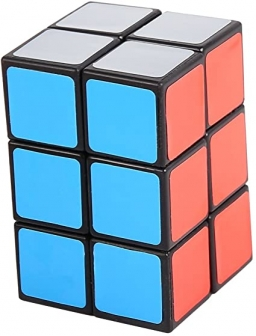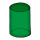# The block

The block, the edges formed by three consecutive GP members, has a surface area of 112 cm2. The sum of the edges that pass through one vertex is 14 cm. Calculate the volume of this block.

V =  64 cm3

### Step-by-step explanation:

Our quadratic equation calculator calculates it.Did you find an error or inaccuracy? Feel free to write us. Thank you!Tips to related online calculators
Looking for help with calculating roots of a quadratic equation?
Do you have a system of equations and looking for calculator system of linear equations?
Tip: Our volume units converter will help you with the conversion of volume units.

## Related math problems and questions:

• Consecutive membersThe block has a volume of 1728 cm³. Determine the lengths of the edges a, b, c of the blocks for which a < b < c and a + b + c = 38 cm and whose numerical values in cm represent three consecutive members of the geometric sequence.
• Block or cuboidThe wall diagonals of the block have sizes of √29cm, √34cm, √13cm. Calculate the surface and volume of the block.
• GP - three membersThe second and third of a geometric progression are 24 and 12(c+1), respectively, given that the sum of the first three terms of progression is 76. determine the value of c.
• Rotary cylinderIn the rotary cylinder it is given: surface S = 96 cm2 and volume V = 192 cm cubic. Calculate its radius and height.
• The cylinderIn a rotating cylinder it is given: the surface of the shell (without bases) S = 96 cm2 and the volume V = 192 cm cubic. Calculate the radius and height of this cylinder.
• Shell area cyThe cylinder has a shell content of 300 cm square, while the height of the cylinder is 12 cm. Calculate the volume of this cylinder.
• Cuboid wallsCalculate the cuboid volume if its different walls have an area of 195cm², 135cm², and 117cm².
• Nice prismCalculate the cuboid's surface if the sum of its edges is a + b + c = 19 cm and the body diagonal size u = 13 cm.
• Cube 1-2-3Calculate the volume and surface area of the cube ABCDEFGH if: a) /AB/ = 4 cm b) perimeter of wall ABCD is 22 cm c) the sum of the lengths of all edges of the cube is 30 cm.
• Cube edgesThe sum of the lengths of the cube edges is 42 cm. Calculate the surface of the cube.
• Hexagonal prism 2The regular hexagonal prism has a surface of 140 cm2 and height of 5 cm. Calculate its volume.
• Cuboid - ratiosThe sizes of the edges of the cuboid are in the ratio 2: 3: 5. The smallest wall has an area of 54 cm2. Calculate the surface area and volume of this cuboid.
• CuboidThe sum of the lengths of the three edges of the cuboid that originate from one vertex is 210 cm. Edge length ratio is 7: 5: 3. Calculate the length of the edges.
• Geometric progressionIn geometric progression, a1 = 7, q = 5. Find the condition for n to sum first n members is sn≤217.
• Cuboid - volume and areasThe cuboid has a volume of 250 cm3, a surface of 250 cm2, and one side 5 cm long. How do I calculate the remaining sides?
• Hard cone problemThe surface of the cone is 200 cm², its height is 7 centimeters. Calculate the volume of this cone.
• Sum of the edgesThe sum of the lengths of all edges of the cube is 72 cm. How many cm2 of colored paper are we going to use for sticking?